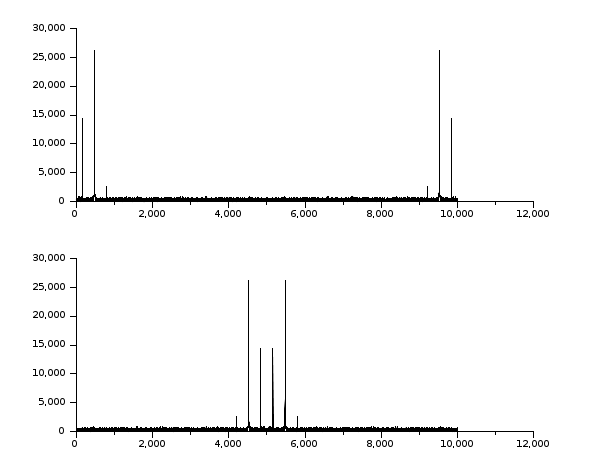Scilab Home page | Wiki | Bug tracker | Forge | Mailing list archives | ATOMS | File exchange
Change language to: English - Português - 日本語 - Русский

Please note that the recommended version of Scilab is 6.0.2. This page might be outdated.
See the recommended documentation of this function

Aide Scilab >> Traitement du Signal > fftshift

fftshift

rearranges the fft output, moving the zero frequency to the center of the spectrum

Calling Sequence

y=fftshift(x [,job])

Arguments

x

real or complex vector or matrix.

y

real or complex vector or matrix.

job

integer, dimension selection, or string 'all'

Description

if x results of an fft computation y= fftshift(x) or y= fftshift(x,"all") moves the zero frequency component to the center of the spectrum, which is sometimes a more convenient form.

If x is a vector of size n, y is the vector x([n/2+1:n,1:n/2])

If x is an m by n matrix y is the matrix x([m/2+1:n,1:m/2],[n/2+1:n,1:n/2]).

[x11 x12]                  [x22 x21]
x=[       ]        gives   y=[       ]
[x21 x22]                  [x12 x11]

y= fftshift(x,n) make the swap only along the nth dimension

Examples

//make a signal
t=0:0.1:1000;
x=3*sin(t)+8*sin(3*t)+0.5*sin(5*t)+3*rand(t);
//compute the fft
y=fft(x,-1);

//display
clf();
subplot(2,1,1);plot2d(abs(y))
subplot(2,1,2);plot2d(fftshift(abs(y)))//make a 2D image
t=0:0.1:30;
x=3*sin(t')*cos(2*t)+8*sin(3*t')*sin(5*t)+..
0.5*sin(5*t')*sin(5*t)+3*rand(t')*rand(t);
//compute the fft
y=fft(x,-1);

//display
clf();
xset('colormap',hotcolormap(256))
subplot(2,1,1);Matplot(abs(y))
subplot(2,1,2);Matplot(fftshift(abs(y)))